Daily 20-Minute Product Tour at 1:00 PM ET

Register Now

# How to Use the 4 Methods of Calculating Depreciation Under US GAAP

December 22, 2022Lease AccountingTo maintain accurate accounting, many companies must follow generally accepted accounting principles (GAAP or US GAAP), which work on the assumption that almost every type of business asset loses value over time. Depreciation allows organizations to account for this lost value and allocate the cost of a tangible asset over its useful life – the estimated lifespan of a depreciable fixed asset, during which it can be expected to contribute to company operations. In accounting, useful life is an important concept since a fixed asset is depreciated over this period of time.

GAAP introduced a set of accounting procedures for asset depreciation to ensure consistency and accuracy among organizations.

## The 4 depreciation methods in accounting

To properly depreciate an asset under GAAP, accounting professionals must calculate the total cost of the asset, how long the asset will last before it must be replaced and how much an asset can sell for at the end of its useful life.

There are four methods for calculating depreciation:

• Straight line method
• Declining balance method
• Units of production method
• Sum-of-the-years’ digits method

Accountants are responsible for figuring out the correct GAAP depreciation method to use based on which method will achieve the most satisfactory allocation of cost. Let’s look more closely at these methods and how businesses can apply them.

### 1. Straight line method

The simplest and most popular method of depreciation is the straight line method. This involves deducting the salvage value from the cost of the asset and dividing the resulting number by the asset’s useful life.

To illustrate this formula, let’s say a company buys a \$15,000 machine with a salvage value of \$4,000 and a useful life of 10 years. If the company in this example used the straight line method of depreciation, the annual depreciation cost would be \$1,100.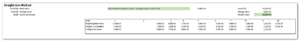### 2. Declining balance method

The declining balance method — a form of accelerated depreciation — allows an organization to depreciate an asset more heavily during its earlier years using a fixed percentage rate. The double declining method is a subset of the declining balance method, but as the name implies, it doubles the rate of depreciation.

These methods are most useful for assets that lose value quickly, such as vehicles, computers, cellphones or other technology.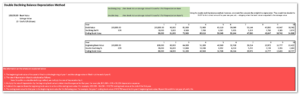### 3. Units of production depreciation method

The formula for the units of production method is similar to that of the straight line method. But instead of using time to define the useful life of an asset, this method uses the number of units produced or hours of operation.

The units of production method allows organizations to deduct higher depreciation costs during years when an asset is used more or produces more units. For example, this may be utilized by a manufacturing company that used a specific piece of machinery to produce X units in 2022 but that will be phased out in 2023 so they will have a lower rate of depreciation next year.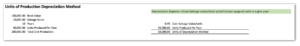### 4. Sum-of-the-years’ digits method

The most complex method is the sum of the years’ digits, which is another form of accelerated depreciation. This method provides a better indication of value for fast-depreciating assets. Because the depreciation formula for the sum of the years’ digits is difficult to calculate, it can present a cumbersome challenge for asset-heavy businesses.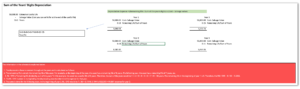Knowing when and how to apply these various depreciation methods in compliance with GAAP can be complicated. Leveraging an innovative software solution such as Visual Lease can help your organization streamline the process, eliminate risk exposure and save more on taxes.### Author Visual Lease

Visual Lease Blogs - read about the best lease administration software, lease management solutions, commercial lease accounting software & IFRS 16 introduction.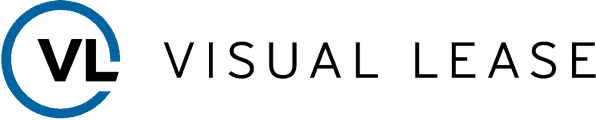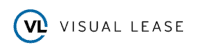Request a Demo